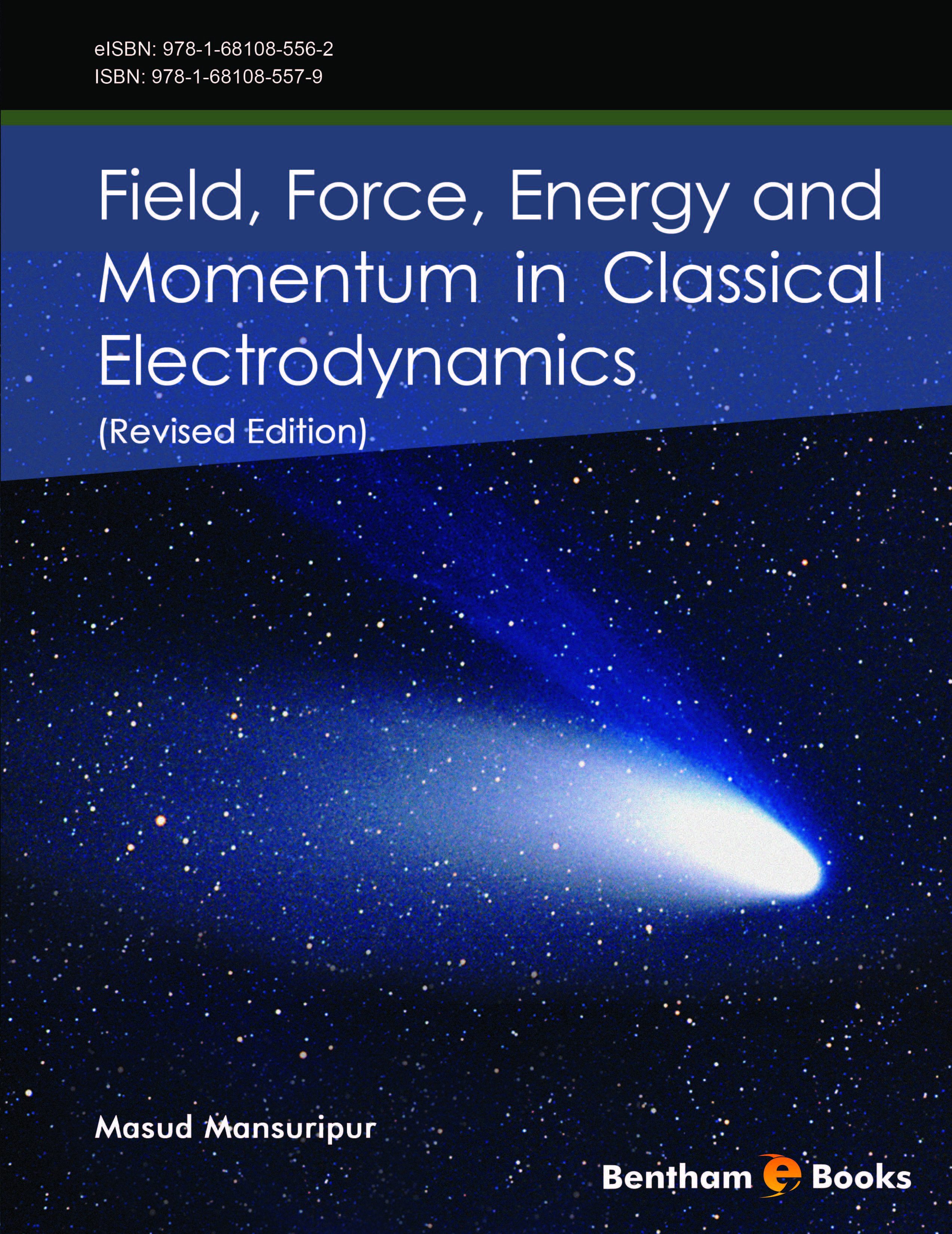Author: Masud Mansuripur

### Field, Force, Energy and Momentum in Classical Electrodynamics (Revised Edition)

eBook: US \$99 Special Offer (PDF + Printed Copy): US \$168
Printed Copy: US \$119
ISBN: 978-1-68108-557-9 (Print)
ISBN: 978-1-68108-556-2 (Online)
Year of Publication: 2017
DOI: 10.2174/97816810855621170101

#### Introduction

The classical theory of electrodynamics is based on Maxwell's equations and the Lorentz law of force. This book begins with a detailed analysis of these equations, and proceeds to examine their far-reaching consequences. The traditional approach to electrodynamics treats the ‘microscopic’ equations of Maxwell as fundamental, with electric charge and electric current as the sole sources of the electric and magnetic fields. Subsequently, polarization and magnetization are introduced into Maxwell's equations to account for the observed behavior of material media. The augmented equations, known as Maxwell's ‘macroscopic’ equations, are considered useful for practical applications, but are also ultimately reducible to the more fundamental ‘microscopic’ equations. In contrast, this textbook treats Maxwell's ‘macroscopic’ equations as the foundation of classical electrodynamics, and treats electrical charge, electrical current, polarization, and magnetization as the basic constituents of material media. The laws that govern the distribution of electromagnetic energy and momentum in space-time are also introduced in an early chapter, then discussed in great detail in subsequent chapters.

The text presents several examples that demonstrate the solution of Maxwell's equations in diverse situations, aiming to enhance the reader’s understanding of the flow of energy and momentum as well as the distribution of force and torque throughout the matter-field systems under consideration.

This revised edition of Field, Force, Energy and Momentum in Classical Electrodynamics features revised chapters, some of which include expanded discussions of fundamental concepts or alternative derivations of important formulas. The new edition also features three additional chapters covering Maxwell’s equations in spherical coordinates (Chapter 10), the author’s recent discussion (and streamlined proof) of the Optical Theorem (Chapter 13), and the fascinating connections between electromagnetism and Einstein’s special theory of relativity (Chapter 15). A new appendix covers the SI system of units that has been used throughout the book.

The book is a useful textbook for physics majors studying classical electrodynamics. It also serves as a reference for industry professionals and academic faculty in the fields of optics and advanced electronics.

# Contributors

##### Author(s):
Masud Mansuripur
College of Optical Sciences
The University of Arizona
Tucson
Arizona